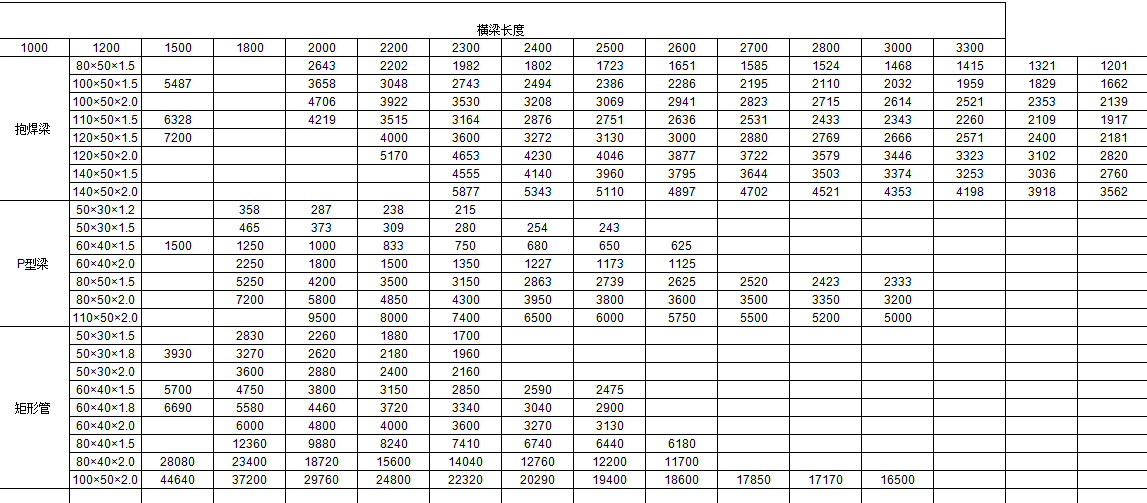News Detail

# Storage shelf carrying capacity calculation

Calculation of shelf Carrying Capacity (unit: kg)1. Calculation of bearing capacity of beam with girdle and rectangular tube section:

The carrying capacity of the shelf is P (total load), the allowable stress of the material of the shelf is [], the length of the beam is L, the modulus of the bending section is WZ. According to the calculation of evenly distributed load of simply supported beam, its bearing capacity is:

For Q235, the allowable stress is 120MPa; for Q215, 105mpa; and for Q195, 90mpa. Therefore, the shelf carrying capacity of the latter two materials should be multiplied by the coefficient of 0.875 and 0.75 respectively on the basis of Q235.

2. P type girder beams bearing capacity calculation: first P type beam cross section centroid calculation, and then calculate IX, IY, IXY, I I Ω Ω X, Y. Calculate the position of bending center X0

Assume transverse bending forces acting on the midpoint of the step. P beam is affected by bending and torsion. The stresses are calculated by bending and torsion respectively, and then the ultimate load is determined by the third strength theory.

The formula for calculating fan-like product of inertias is:

In the formula: if, then take:.

The torque of P beam is the maximum at the beam end and the minimum at the span, which is close to zero. Therefore, according to approximate calculation, the bending strength can be calculated in the middle of the span and the torsion strength can be calculated at the support end respectively. Maximum torque: QLx0/2.

Or MT is equal to px0/2. Because X0 < < l. So the maximum torque is much less than the maximum bending moment (PL/8). Because the P beam is a closed thin-walled beam. So the torsional shear stress is much less than the flexural normal stress.

So the ultimate load is calculated according to the bending normal stress. But times the conversion factor of 0.6.

The calculation of p-type pipe is based on the hypothesis of closed thin-walled pipe. If it is an open thin-walled pipe (pipe longitudinal is not completely welded), its carrying capacity is only 1/3~1/4 of the calculated value.